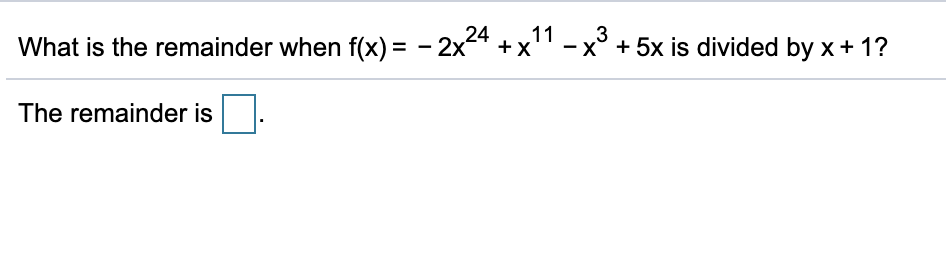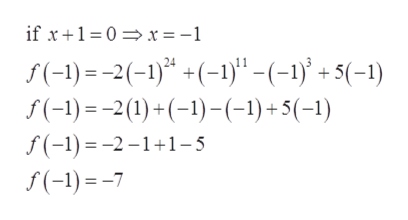# 113- x° + 5x is divided by x + 1?What is the remainder when f(x) = - 2x4 + x'The remainder is

Question
1 viewshelp_outlineImage Transcriptionclose11 3 - x° + 5x is divided by x + 1? What is the remainder when f(x) = - 2x4 + x' The remainder is fullscreen
check_circle

Step 1

Find the reminder when

Step 2

Using reminder ...help_outlineImage Transcriptioncloseif x+1=0 =x = -1 f(-1) = -2(-1)* +(-1)" -(-1)' + 5(-1) S(-1) =-2(1) +(-1)-(-1)+5(-1) f(-1) = -2 –1+1-5 f(-1) =-7 fullscreen

### Want to see the full answer?

See Solution

#### Want to see this answer and more?

Solutions are written by subject experts who are available 24/7. Questions are typically answered within 1 hour.*

See Solution
*Response times may vary by subject and question.
Tagged in

### Other